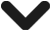# Accounting vs Economic Profit

Table of Contents# Accounting vs Economic Profit

## What is Profit

Profit refers to the money a firm makes after all its costs are considered. It is calculated by taking the firms revenue (the cash it receives from its sales) and subtracting away its costs. These include the cost of its employees, rent, equipment, maintenance, loan payments, taxes, and many more. Only once these costs have been taken away do we end up with the firms underlying profit.

There are two main types of profit. Accounting profit is the most commonly used in finance and is frequently reported in firms annual reports. There is also economic profit, which is used more as a way of highlighting opportunity costs between options. This factors in both implicit and explicit costs so allows individuals and firms to consider the opportunity costs they are incurring when choosing one option over another.

##### Key Points
1. Profit is the amount a firm makes when it subtracts its total costs from its revenue.
2. Accounting profits are profits which account for explicit costs such as rent and utilities, whilst economic profits also subtract the implicit costs – the cost of choosing one decision over another.

## Explicit and Implicit Costs

Explicit and implicit costs are both important aspects in determining a firms accounting and economic profit. An explicit cost is one by which is a clear monetary amount which the firm incurs as a result of its daily activities. It is a clear and visible cost that the firm incurs and is incorporated onto its balance sheet. Examples include rent, wages, supplies, and utilities. It is common for this to be used to find the firms accounting profit which is provided to shareholders.

By contrast, implicit costs are costs which are not visible and are incurred due to choosing one option over another. This can also be seen as the opportunity cost. For instance, choosing to go to University creates an explicit cost of paying for tuiton as well as accomodation. However, there is also the implicit cost – which is the money a student could have made had they been working instead.

## Accounting Profit

A firm’s accounting profit is the money it has made from sales, minus its total explicit costs. This may include costs such as employees wages, rent, utility bills, loan repayments, and other day to day expenditures.

It is known as accounting profit as this is what is accounted for in the firms financial statements to shareholders. These are what the firm has explicitly spent on its activities and therefore the profits or losses, and expenditures are all accounted for on the statement.

Examples include:

1. Labor costs, such as wages.
2. Inventory needed for production.
3. Raw materials.
4. Transportation costs.
5. Sales and marketing costs.

## Economic Profit

A firm’s economic profit includes all the revenue it made, minus both explicit and implicit costs. In other words, it includes everything in accounting profit, but also includes the value of opportunity costs. Also known as implicit costs, these are those which cost the company had they chosen a different option.

For example, a firm may invest \$1 million in a new factory. That is the explicit cost. However, it could potentially earn \$20,000 in interest alone. So the economic profit also factors in the alternative use for that resource. This would mean that totally economic profits will be lower than accounting profits as they also consider the cost of foregoing an alternative use.

## Accounting Profit vs Economic Profit

The main difference between accounting and economic profit is that economic profit includes implicit costs. Whilst accounting profits include the raw costs of doing business, economic profit includes the opportunity cost of employing those resources for an alternative use.

For example, a firm may spend \$10,000 training 10 of its employees over a week. That is the explicit cost and is factored into the accounting profit. However, there is also the implicit cost. That is the weeks lost labor from those employees. This may cost the firm \$5,000 of lost output. So the total economic cost is the explicit cost of the training, plus the implicit cost of the lost labour. So in total, there is an economic cost of \$15,000. It is this amount that is subtracted from the firms revenue.

## FAQs on Accounting and Economic Profit

Why is economic profit better than accounting profit?

Economic profit is a useful indicator to businesses as it shows the impact decisions make on the firms bottom line. If one year the firm makes more money, it may mean better decisions were taken. Similarly, the firm may be making an accounting profit, but its economic profit may be negative. This provides the businesses with valuable insight that may suggest the firm isn’t operating as efficiently as it could.

What is an example of accounting profit?

Accounting profit is calculated by taking a firms revenue and subtracting the firms explicit costs. This includes anything that is tangible and quantifiable such as wages, utilities, and rent.

What is the difference between economic profit and accounting profit?

The main difference between economic profit and accounting profit is that economic profit factors in opportunity cost. Whilst accounting profit subtracts explicit costs such as rent, utilities, and wages from revenue – economic profit also subtracts implicit costs which are the opportunity costs to the firm from choosing Option A over Option B.Nash Equilibrium: Definition & Examples - Nash equilibrium refers to the situation whereby a group of individuals choose the most optimal strategy and do not deviate…Income Effect Definition - The income effect is where a change in income has a subsequent effect on demand. In other words, as consumers…